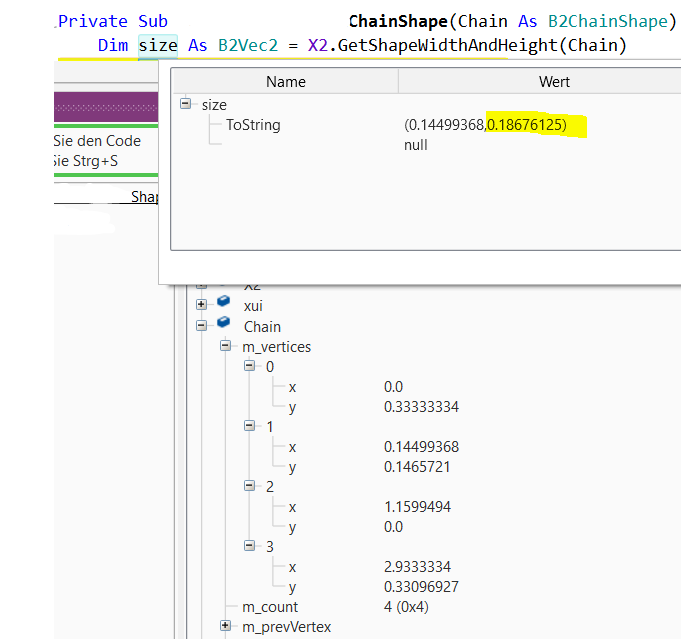GamesStrange Values returned by GetShapeWidthAndHeight<->ChainShape

Gunther

Active Member
Please have a look to the sizing values of a ChainType Shape.

It seems that the Shape Width and Height are not correct returned by the function.

The X value is the one from the first Edge vertice and the Y value is a moon value.

According to the actual values in that chain is should be something like ( 5.96 , 0.66 ).

The chain values are read via Tiled into the app.

Possible bug or not the best approach of mine?Erel

B4X founder
Staff member
GetShapeAndWidth shouldn't be used with chain shapes. Only with polygon, rectangle or circle shapes.

•Gunther

Gunther

Active Member
Ok, how I get savely the dimentions then for a chain shape?

Erel

B4X founder
Staff member
Untested code:
B4X:
Dim edge As B2EdgeShape
edge.Initialize(Null, Null)
chain.GetEdge(0, edge)
Dim BottomLeft, TopRight As B2Vec2
BottomLeft.X = Min(edge.Vertex1.X, edge.Vertex2.X)
TopRight.X = Max(edge.Vertex1.X, edge.Vertex2.X)
BottomLeft.Y = Min(edge.Vertex1.Y, edge.Vertex2.Y)
TopRight.Y = Max(edge.Vertex1.Y, edge.Vertex2.Y)
Dim aabb As B2AABB
aabb.Initialize2(BottomLeft, TopRight)
For i = 1 To chain.EdgeCount - 1
chain.GetEdge(i, edge)
aabb.BottomLeft.X = Min(aabb.BottomLeft.X, Min(edge.Vertex1.X, edge.Vertex2.X))
aabb.TopRight.X = Max(aabb.TopRight.X, Max(edge.Vertex1.X, edge.Vertex2.X))
aabb.BottomLeft.Y = Min(aabb.BottomLeft.Y, Min(edge.Vertex1.Y, edge.Vertex2.Y))
aabb.TopRight.Y = Max(aabb.TopRight.Y, Max(edge.Vertex1.Y, edge.Vertex2.Y))
Next
aabb.Width and Height store the width and height.

Android Code Snippet [B4X] Round clipping panel
Replies
2
Views
2K
Replies
11
Views
4K
Replies
9
Views
1K
Replies
8
Views
9K
Replies
0
Views
2K